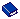Function to Retrieve Rate Amounts for Exports

#### Description

This function calculates a rate amount based on the specified input parameters for the export date. It may be used as a formula when configuring the column details for an export.

This function internally calls pkg_rate_price.get_daily_rate_summary() so that the retrieved amount is the same as that displayed on the Rate Info screen in PMS.

The function pkg_rate_price.get_daily_rate_summary() returns a PL/SQL table with one rate amount per day (depending on the Number of Nights parameter). The records in the PL/SQL table have three amount columns:

R_AMOUNT = room revenue amount

P_AMOUNT = package amount

T_AMOUNT = tax amount

The get_rate_amount function will always return the R_AMOUNT. If parameter Include Packages YN is 'Y' then the P_AMOUNT will be added and in case parameter Include Taxes YN is 'Y' the T_AMOUNT will be added as well.

#### Function

exp_gen.get_rate_amount()

#### Parameters

 Parameter Description Rate Code Name Rate code for which amount is to be calculated. Room Type Label Room type for which amount is to be calculated. Include Packages YN Should packages be included? Y or N. Default = N. Include Taxes YN Should taxes be included? Default = N. Arrival Date Arrival date. Default = pms_p.business_date. Number of Nights Nights included in stay. Default = 1. If the Number of Nights is greater than 1, the function will add together the rate amounts for the number of nights. Resort Resort ID. Default = pms_p.resort Number of Adults Adults on reservation. Default = 1. Number of Children Children on reservation. Default = 0. If any of the Number of Children in Age Group parameters are used, they will overwrite any Number of Children value. Number of Children in Age Group 1 Where Child Age Buckets are used, the number of children in age bucket 1. Default = 0. Number of Children in Age Group 2 Where Child Age Buckets are used, the number of children in age bucket 2. Default = 0. Number of Children in Age Group 3 Where Child Age Buckets are used, the number of children in age bucket 3. Default = 0.

#### Example

Assume that the Rate Info for the rate code AA for the room type DLX displays the following amounts:

Room Revenue: 2017

Packages: 20

Generates (Taxes): 145.19

When the function is executed with the parameters shown

` exp_gen.get_rate_amount('AA','DLX','Y','Y',pms_p.business_date,1);`

The result is 2182.19

The results are as follows with the noted parameter variations:

Changed parameters to `exp_gen.get_rate_amount('AA','DLX','N','N');` - Result 2017

Changed parameters to `exp_gen.get_rate_amount('AA','DLX','Y','N');` - Result 2037

Changed parameters to `exp_gen.get_rate_amount('AA','DLX','N','Y'); ` - Result 2162.19